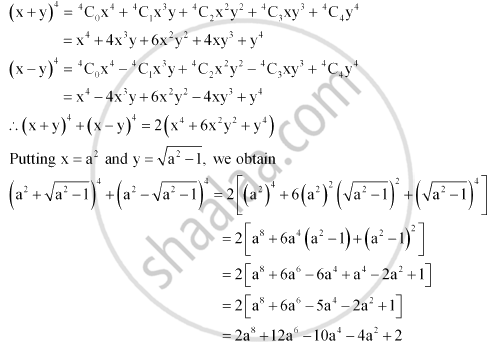Share

# Find the Value of (A^2 + Sqrt(A^2 - 1))^4 + (A^2 - Sqrt(A^2 -1))^4 - Mathematics

#### Question

Find the value of (a^2 + sqrt(a^2 - 1))^4 + (a^2 - sqrt(a^2 -1))^4

#### Solution

Firstly, the expression (x + y)4 + (x – y)4 is simplified by using Binomial Theorem.

This can be done asIs there an error in this question or solution?